# Solution assignment 02 Tangent line to graph

### Assignment 2

Calculate the slope of the tangent line at the point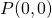of the graph of the function: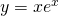### Solution

First we calculate the derivative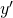of the function  (using the product rule):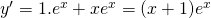In the point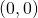the derivative has the value: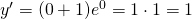and thus the slope of the tangent line atequals.